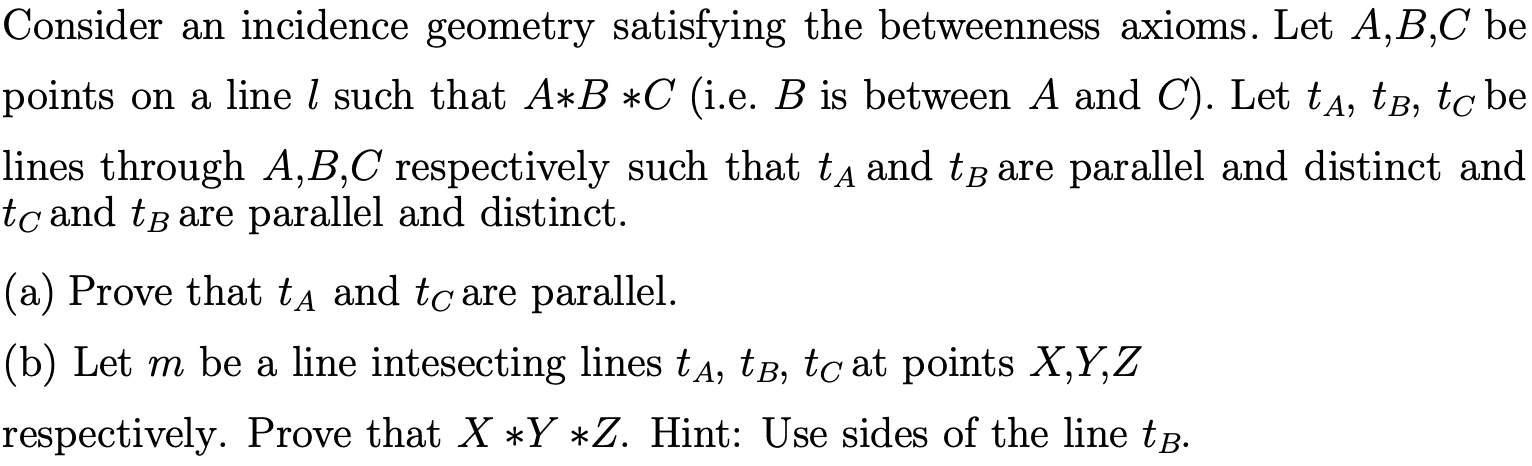# Consider an incidence geometry satisfying the betweenness axioms. Let A,B,C bepoints on a line / such that A*B *C (i.e. B is between A and C). Let tA, tB, to belines through A,B,C respectively such that tA and tB are parallel and distinct andtc and tB are parallel and distinct|(a) Prove that tA and tc are parallel.(b) Let m be a line intesecting lines tA, tB, tc at points X,Y,Zrespectively. Prove that X Y *Z. Hint: Use sides of the line tB.

Question
26 viewshelp_outlineImage TranscriptioncloseConsider an incidence geometry satisfying the betweenness axioms. Let A,B,C be points on a line / such that A*B *C (i.e. B is between A and C). Let tA, tB, to be lines through A,B,C respectively such that tA and tB are parallel and distinct and tc and tB are parallel and distinct |(a) Prove that tA and tc are parallel. (b) Let m be a line intesecting lines tA, tB, tc at points X,Y,Z respectively. Prove that X Y *Z. Hint: Use sides of the line tB. fullscreen
check_circle

Step 1

Given ta is parallel to tb and tb is parallel to tc

Step 2

(a)To prove: ta and tc are parallel

Proof:

Let θ1, θ2 and θ3 be the angle shown in the figure.

When two lines are parallel, their respective corresponding angles are equal.

So here θ1= θ2 and θ2= θ3

It can be concluded that θ1= θ2= θ3

Which implies θ1 = θ3

Which implies ta and tc are parallel.

Step 3

To prove: X*Y*Z

Given m be a line intersecting the line ta, tb and tc at the point X...

### Want to see the full answer?

See Solution

#### Want to see this answer and more?

Solutions are written by subject experts who are available 24/7. Questions are typically answered within 1 hour.*

See Solution
*Response times may vary by subject and question.
Tagged in